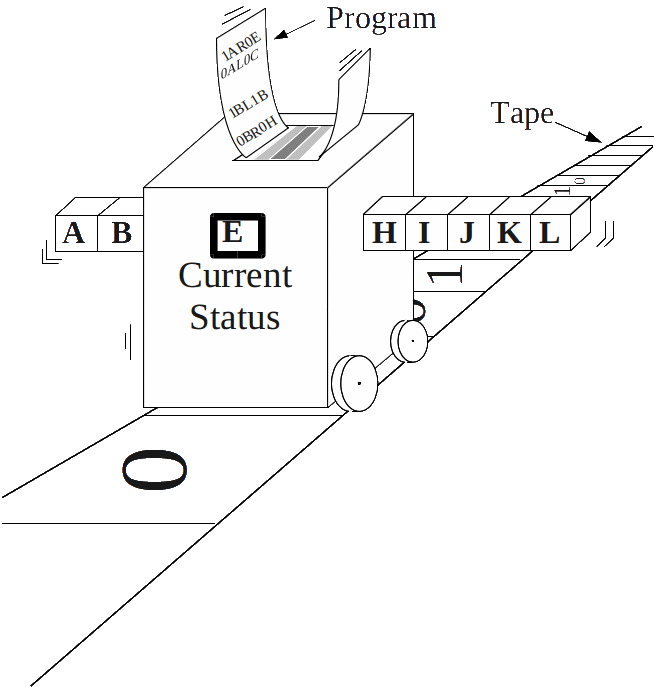## Discrete ComputabilityDiscrete Computability is the theory of the capabilities and limitations of (at least) four entirely distinct notions of effectiveness:

• Turing machines
• lambda calculus
• $\mu$ recursion
• WHILE programs

Surprisingly, they all have turned out as equivalent: which is considered as strong evidence of this being indeed a sensible and natural notion.

Formally, a language (also called a decision problem) is a subset $L$ of either the integers $\mathbb{N}$ or the set $\{0,1\}^*$ of finite binary strings. It is decidable (or recursive) if a Turing machine can, upon input of $x$, within finite time (terminate and) determine whether $x$ belongs to $L$ or not. $L$ is semi-decidable if a Turing machine can terminate on inputs $x \in L$ and diverge for $x \notin L$. $L$ is recursively enumerable (commonly abbreviated as `r.e.') if a Turing machine is able to output in arbitrary order all elements of $L$. If the complement of $L$ is r.e., $L$ is called co-r.e.

With the important technique of dove-tailing (basically the interleaved simulation of countably many computations), semi-decidability can be shown to be equivalent to recursive enumerability; and recursiveness to both r.e. and co-r.e.

Another crucial ingredient is an effective enumeration of all Turingmachines: there exists a universal Turing machine $U$ which, given an encoding of a Turingmachine $M$ and input $x$, can simulate $M$ on $x$.

The code $\langle M\rangle$ of $M$ is called its Gödel index the mapping $M\mapsto\langle M\rangle$ a Gödelization. Several codes and inputs may be combined into, and extracted back from, a single integer by virtue of a pairing function like for instance \begin{equation} \mathbb{N}\times\mathbb{N} \;\ni\; (x,y)\;\mapsto\;\langle x,y\rangle \;:=\; 2^x\cdot (2y+1) \enspace . \end{equation}

A given machine $M$ and input $x$ can be recoded into some $M'$ which already contains $x$ and, more precisely, ignores its own input $x'$ but always behaves like $M$ on $x$. Slightly more generally, the SMN Theorem asserts that any (code of a) Turingmachine realizing a partial function $(x,y)\mapsto f(x,y)$ and a given $x_0$ may be converted into (the code of) a machine realizing $y\mapsto f(x_0,y)$. The reverse conversion is known as the UTM Theorem, namely from a mapping $x\mapsto\langle M_x\rangle$ to the mapping $\langle x,y\mapsto f_x(y)$, where $f_x$ denotes the partial function realized by Turingmachine $M_x$. SMN and UTM together permit effective adaptation of the arity of functions under consideration and are sometimes referred to as type conversion. They also imply that a Gödelization must enumerate every computable function infinitely often: $f_{x_1}=f_{x_2}=\ldots$ for some increasing sequence $x_1,x_2,\ldots$.

Now suppose that the Halting problem \begin{equation} H \quad=\quad \big\{ \langle M,x\rangle : M \text{ terminates on input }x\big\} \end{equation} were decidable. Its complement could then be semi-decided. This in turn would imply that the diagonal problem \begin{equation} D \quad=\quad \big\{\langle M\rangle: M \text{ does not terminate on }\langle M\rangle\big\} \end{equation} could be accepted by some machine $M_0$. Now either $\langle M_0\rangle\in D$ or $\langle M_0\rangle\notin D$ holds. In the first case, by the definition of $D$, $M_0$ does not terminate on input $\langle M_0\rangle$—contradicting that $M_0$ accepts $D$, i.e. terminates exactly on inputs from $D$ like $\langle M_0\rangle$. Similarly, the second case $\langle M_0\rangle\notin D$ means divergence of $M_0$ on input $\langle M_0\rangle$ and implies $\langle M_0\rangle\in D$: again, this is a contradiction. $\Box$

The restricted (and thus seemingly easier) problem $\big\{\langle M\rangle : M \text{ terminates on empty input}\big\}$ is sometimes also referred to as $H$. In fact each can be reduced to the other by virtue of the UTM theorem and are thus equally undecidable.

### Applications

The easy part of the Halting Problem is of course verifying that a Turingmachine will halt; $H$ is semi-decidable. The difficulty lies in identifying that a machine will continue running forever—which one can never be sure of within finite time. Were that possible, many important open mathematical claims (Goldbach Conjecture, Riemann Hypothesisetc.) could be settled just by setting up a Turingmachine $M$ to look for a counter example and checking whether this search eventually succeeds, that is, whether $M$ terminates.

One generally expects that these open questions may ultimately be solved without having to resort to the Halting problem but by mathematical reasonings (and the insights they provide). This has been achieved, e.g. for Fermat's Last Theorem. However, according to Rice's Theorem, many other problems can provably be solved only in connection with $H$. Automated software verification, for instance, as desirable as it may be, is principally infeasible in that even the simplest requirement of correct software—termination upon empty input—cannot be decided algorithmically.

Since Turing's start, a rich variety of further problems has been proven undecidable; to mention two rather famous ones:

• the Word Proble for finitely presented groups;
• the integral solvability of Diophantine Equations (also known as Hilbert's Tenth Problem).
These exhibit serious principal limitations of computational group theory and number theory. A positive result in computability theory has recently been awarded the Gödel Prize 2002:
• The equivalence of linear pushdown automata can be decided by a Turing machine.

The above considerations refer to decision problems, that is, questions (encoded as integers or binary strings) to be answered with yes or no. A seemingly more general type of problem involves integers or binary strings also as output, that is, the evaluation of a (possibly partial) function. On the other hand this problem turns out equivalent to the recursive enumerability of the function's graph: a decision problem.

Selected References:

• Moret: The Theory of Computation (1998).
• Lewis, Papadimitriou: Elements of the Theory of Computation, Prentice-Hall (1997).
• Soare: Computability Theory and Applications (2016).
• Senizergues: The equivalence problem for deterministic pushdown automata is decidable (2005).